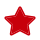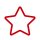# sum

#### 函数原型

te.lang.cce.sum(raw_tensor, axis, keepdims=False)

#### 参数说明

• raw_tensor：输入tensor，tvm.tensor类型
• axis：做reduce操作的轴，取值范围：[-d,d-1]，其中d是raw_tensor的维数，int或list类型
• keepdims：默认值是False，表示做reduce操作后，操作的轴长度为0，例如，原shape是(10,10,10)，keepdims=False时，reduce后shape是(10,10)。若将该参数值设置为True，表示做reduce操作后，操作的轴的长度设置为1，例如，原shape是(10,10,10)，keepdims=True时，reduce后shape是(10,10,1)。

#### 返回值

res_tensor：结果tensor，tvm.tensor类型

#### 调用示例

```shape = (1024,1024)
input_dtype = "float16"
data = tvm.placeholder(shape, name="data", dtype=input_dtype)
res = te.lang.cce.sum(data, axis=1)```

# 相关产品